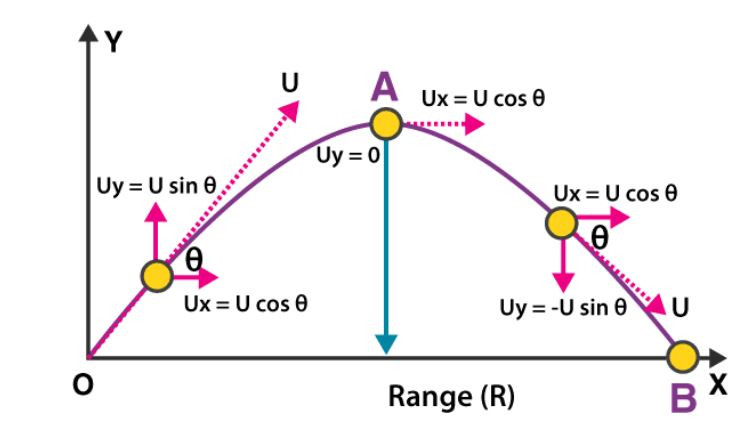Search

# Projectile Motion

## What is Projectile?

A projectile is any object thrown into space upon which the only acting force is gravity. The primary force acting on a projectile is gravity. This doesn’t necessarily mean that other forces do not act on it, just that their effect is minimal compared to gravity. The path followed by a projectile is known as a trajectory. A baseball batted or thrown is an example of the projectile.

What is Projectile Motion? When a particle is thrown obliquely near the earth’s surface, it moves along a curved path under constant acceleration that is directed towards the centre of the earth (we assume that the particle remains close to the surface of the earth). The path of such a particle is called a projectile and the motion is called projectile motion. In a Projectile Motion, there are two simultaneous independent rectilinear motions:

1. Along the x-axis: uniform velocity, responsible for the horizontal (forward) motion of the particle.

2. Along y-axis: uniform acceleration, responsible for the vertical (downwards) motion of the particle.

Acceleration in the horizontal projectile motion and vertical projectile motion of a particle: When a particle is projected in the air with some speed, the only force acting on it during its time in the air is the acceleration due to gravity (g). This acceleration acts vertically downward. There is no acceleration in the horizontal direction, which means that the velocity of the particle in the horizontal direction remains constant. Parabolic Motion of Projectiles Let us consider a ball projected at an angle θ with respect to the horizontal x-axis with the initial velocity u as shown below:The point O is called the point of projection; θ is the angle of projection and OB= Horizontal Range or Simply Range. The total time taken by the particle from reaching O to B is called the time of flight.